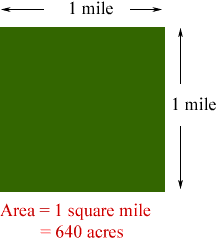Quandaries and Queries Who is asking: Student Level: Middle Question: How many acres are in 1 mile? If there are 12 acres how many miles would that be,would that be even a mile? Hi, Miles and acres measure entirely different things. Miles are units of length and acres are units of area. There is a connection between acres and SQUARE miles. If you think of a piece of land in the shape of a square and measures one mile on each side then its area is one square mile. In terms of acres the area is 640 acres.Penny Go to Math Central